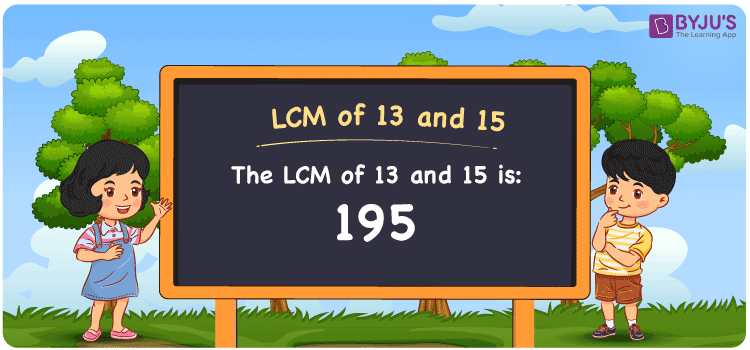Checkout JEE MAINS 2022 Question Paper Analysis : Checkout JEE MAINS 2022 Question Paper Analysis :

# LCM of 13 and 15

LCM of 13 and 15 is 195. The value evenly divisible by the numbers 13 and 15 gives the LCM. The Least common multiple of 13 and 15 can be found from the multiples which are common in the given numbers. The multiples of 13 are (13, 26, 39, 52, ….) and the multiples of 15 are (15, 30, 45, 60, 75, 90, 105, ….) respectively. Prime factorisation, listing of the multiples and division are the few methods used to find the LCM.

## What is LCM of 13 and 15?

The answer to this question is 195. The LCM of 13 and 15 using various methods is shown in this article for your reference. The LCM of two non-zero integers, 13 and 15, is the smallest positive integer 195 which is divisible by both 13 and 15 with no remainder.## How to Find LCM of 13 and 15?

LCM of 13 and 15 can be found using three methods:

• Prime Factorisation
• Division method
• Listing the multiples

### LCM of 13 and 15 Using Prime Factorisation Method

The prime factorisation of 13 and 15, respectively, is given by:

13 = 13¹

15 = 3 x 5 = 3¹ x 5¹

LCM (13, 15) = 195

### LCM of 13 and 15 Using Division Method

We’ll divide the numbers (13, 15) by their prime factors to get the LCM of 13 and 15 using the division method (preferably common). The LCM of 13 and 15 is calculated by multiplying these divisors.

 3 13 15 5 13 5 13 1 1 x 1 1

No further division can be done.

Hence, LCM (13, 15) = 195

### LCM of 13 and 15 Using Listing the Multiples

To calculate the LCM of 13 and 15 by listing out the common multiples, list the multiples as shown below

 Multiples of 13 Multiples of 15 13 15 26 30 39 45 52 60 65 75 78 90 91 105 104 120 117 135 130 150 143 165 156 180 169 195 182 210 195 225 208 240

LCM (13, 15) = 195

## Video Lesson on Applications of LCM## LCM of 13 and 15 Solved Examples

If the LCM and GCD of two numbers are 195 and 1 respectively, calculate the other number if one number is 15.

Solution:

Consider the number as z

We know that

GCD x LCM = 15 x z

z = (GCD x LCM)/15

Substituting the values

z = (195 x 1)/15

z = 13

Hence, the other number is 13.

## Frequently Asked Questions on LCM of 13 and 15

### What is the LCM of 13 and 15?

The LCM of 13 and 15 is 195.

### Find GCF if the LCM of 13 and 15 is 195.

LCM x GCF = 13 x 15

Given

LCM of 13 and 15 is 195

195 x GCF = 195

GCF = 195/195 = 1

### What are the methods used to find the LCM of 13 and 15?

The methods used to find the LCM of 13 and 15 are Prime Factorisation, Division method and Listing the multiples